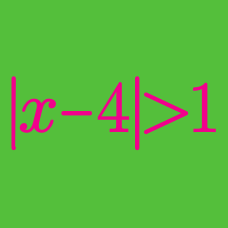Algebra

Absolute Value Inequalities: Level 3 Challenges

$\left | 1 - \frac{|x|}{1 + |x|} \right| \geq \frac{1}{2}$

The solution set to this inequality is $a \le x \le b.$ What is $b-a?$

How many integers $x$ satisfy the inequality $|x-2000|+|x| \leq 9999$?

How many ordered pairs of integers $(x,y)$ are there that satisfy $|x| + |y| \leq 10$?

Consider all monic polynomials $f(x) = x^2 + bx + c$, where $b$ and $c$ are real numbers. What is the minimum value of $N$, where

$N = \max_{x \in [-10,10]} \vert f(x) \vert?$

Details and assumptions

The last equation states: "The maximum value of the absolute value of $f(x)$ , as $x$ ranges from $-10$ to $10$ inclusive".

$\large 2=|x-2|+|x-4|$

Find the solution set of the above equation.

×